# Discrete-Time Integrator

•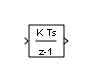HDL Coder / Discrete
HDL Coder / HDL Floating Point Operations

## 描述

• 在模块对话框上定义初始条件，或作为模块的输入

• 定义输入增益 (K) 值

• 输出模块状态

• 定义积分的上限和下限

• 使用其他重置输入重置状态

### 输出方程

• 前向欧拉方法：

`y(n) = y(n-1) + K*[t(n) - t(n-1)]*u(n-1)`
• 后向欧拉方法：

`y(n) = y(n-1) + K*[t(n) - t(n-1)]*u(n)`
• 梯形法：

`y(n) = y(n-1) + K*[t(n)-t(n-1)]*[u(n)+u(n-1)]/2`

Simulink 根据模块的采样时间（可以是显式或触发的采样时间）自动选择这些输出方程的状态空间实现。当使用显式采样时间时，对于所有 `n > 0` 的步长，`t(n)-t(n-1)` 将减小到采样时间 `T`

### 积分方法和累积方法

```x(n+1) = x(n) + K*T*u(n) y(n) = x(n)```

```Step 0: y(0) = IC (clip if necessary) x(1) = y(0) + K*T*u(0) Step 1: y(1) = x(1) x(2) = x(1) + K*T*u(1) Step n: y(n) = x(n) x(n+1) = x(n) + K*T*u(n) (clip if necessary)```

`y(n) = y(n-1) + K*T*u(n).`

• 如果触发子系统和函数调用子系统的参数初始条件设置设置为“`输出`”或“`自动`”：

```Step 0: y(0) = IC (clipped if necessary) x(1) = y(0)```
• 如果非触发子系统的参数初始条件设置设置为 “”：

```Step 0: x(0) = IC (clipped if necessary) x(1) = y(0) = x(0) + K*T*u(0) Step 1: y(1) = x(1) + K*T*u(1) x(2) = y(1) Step n: y(n) = x(n) + K*T*u(n) x(n+1) = y(n)```

`T` 固定时（等于采样周期），计算输出的表达式为：

```x(n) = y(n-1) + K*T/2*u(n-1) y(n) = x(n) + K*T/2*u(n)```
• 如果触发子系统和函数调用子系统的参数初始条件设置设置为“`输出`”或“`自动`”：

```Step 0: y(0) = IC (clipped if necessary) x(1) = y(0) + K*T/2*u(0)```
• 如果非触发子系统的参数初始条件设置设置为 “”：

```Step 0: x(0) = IC (clipped if necessary) y(0) = x(0) + K*T/2*u(0) x(1) = y(0) + K*T/2*u(0) Step 1: y(1) = x(1) + K*T/2*u(1) x(2) = y(1) + K*T/2*u(1) Step n: y(n) = x(n) + K*T/2*u(n) x(n+1) = y(n) + K*T/2*u(n)```

`T` 是变量时（例如，从触发时间获得），模块将按照以下时间步计算输出。

• 如果触发子系统和函数调用子系统的参数初始条件设置设置为“`输出`”或“`自动`”：

```Step 0: y(0) = IC (clipped if necessary) x(1) = y(0)```
• 如果非触发子系统的参数初始条件设置设置为 “”：

```Step 0: x(0) = IC (clipped if necessary) x(1) = y(0) = x(0) + K*T/2*u(0) Step 1: y(1) = x(1) + K*T/2*(u(1) + u(0)) x(2) = y(1) Step n: y(n) = x(n) + K*T/2*(u(n) + u(n-1)) x(n+1) = y(n)```

### 定义初始条件

• 要将初始条件定义为模块参数，请将初始条件来源参数设置为 `internal` 并在初始条件文本框中输入值。

• 要从外部源提供初始条件，请将初始条件来源参数设置为 `external`。模块上出现一个额外的输入端口。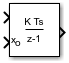### 何时使用状态端口

• 当模块输出通过重置端口或初始条件端口馈送回模块，从而产生代数环时。有关示例，请参阅 `sldemo_bounce_two_integrators` 模型。

• 当您要从一个条件执行子系统向另一个条件执行子系统传递状态，从而可能导致计时问题时。有关示例，请参阅Building a Clutch Lock-Up Model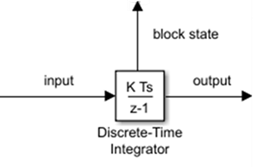### 限制积分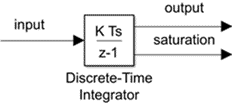• 1 表示正在应用上限。

• 0 表示积分不受限制。

• -1 表示正在应用下限。

### 重置状态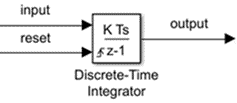### 重置触发器类型

• `上升沿`” - 当重置信号具有上升沿时重置状态。例如，下图显示了上升沿重置触发器对后向欧拉积分的影响。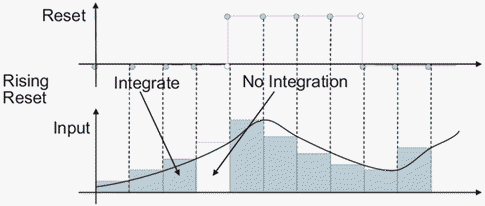• `下降沿`” - 当重置信号具有下降沿时重置状态。例如，下图显示了下降沿重置触发器对后向欧拉积分的影响。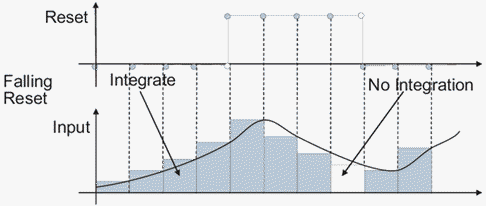• `任一沿`” - 当重置信号上升或下降时重置状态。例如，下图显示了任一沿重置触发器对后向欧拉积分的影响。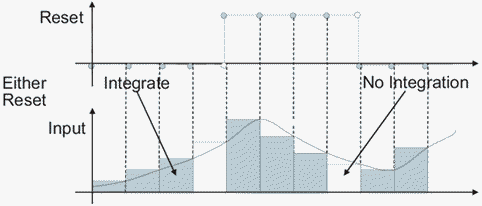• `电平`” - 当重置信号非零时重置输出并使输出保持在初始条件。例如，下图显示了电平重置触发器对后向欧拉积分的影响。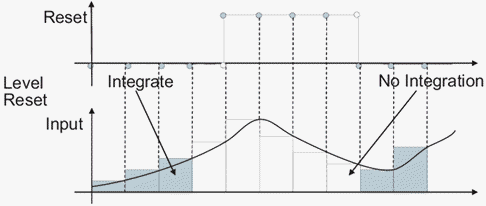• `采样电平`” - 当重置信号非零时，将输出重置为初始条件。例如，下图显示了采样电平重置触发器对后向欧拉积分的影响。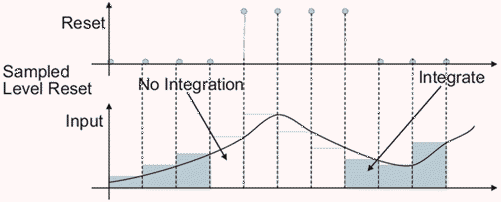`采样电平`”重置选项需要的计算比较少，因此效率比“`电平`”重置选项高。

注意

对于 Discrete-Time Integrator 模块，所有触发器检测都基于具有正值的信号。例如，从 -1 变成 0 的信号不是上升沿，但从 0 变成 1 的信号是上升沿。

### 简化初始化模式下的行为

• 在代数环中

• 在启用和禁用时

• 在比较使用触发采样时间和使用显式采样时间（触发模块的速率与显式采样时间的速率相同）的结果时

“初始条件设置”设置为“输出”时的启用和禁用行为

``` y(td) = y(td-1) ```

• 如果父级子系统控制端口的启用时的状态设置为“`重置`”：

`y(te) = IC.`
• 如果父级子系统控制端口的启用时的状态设置为“`保持`”：

`y(te) = y(td).`

下图显示了这种情况。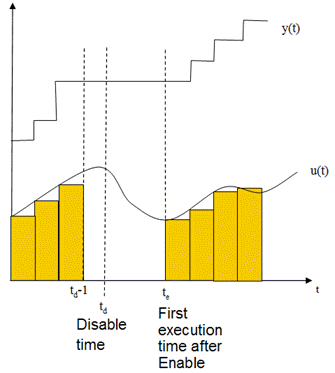### 函数调用子系统内的使能子系统中的行为

`t — tstart`

`t — tprevious`

## 端口

### 输出

• `1` 表示正在应用上限。

• `0` 表示积分不受限制。

• `-1` 表示正在应用下限。

#### 依存关系

• 模块输出通过重置端口或初始条件端口馈送回模块，从而产生代数环。有关示例，请参阅 `sldemo_bounce_two_integrators` 模型。

• 您要从一个条件执行子系统向另一个条件执行子系统传递状态，从而可能导致计时问题。有关示例，请参阅 `sldemo_clutch` 模型。

## 参数

### 常设

#### 编程用法

 模块参数：`IntegratorMethod` 类型：字符向量 值：`'Integration: Forward Euler'` | `'Integration: Backward Euler'` | `'Integration: Trapezoidal'` | `'Accumulation: Forward Euler'` | `'Accumulation: Backward Euler'` | `'Accumulation: Trapezoidal'` 默认值：`'Integration: Forward Euler'`

• 指定除 1.0（默认值）之外的值在语义上等效于将 Gain 模块连接到积分器的输入。

• 有效值包括：

• `double(1.0)`

• `single(1.0)`

• `[1.1 2.2 3.3 4.4]`

• `[1.1 2.2; 3.3 4.4]`

#### 编程用法

 模块参数：`gainval` 类型：字符向量 值：标量 | 向量 默认值：`'1.0'`

• `无`” - 不将状态重置为初始条件。

• `上升沿`” - 当重置信号具有上升沿时重置状态。

• `下降沿`” - 当重置信号具有下降沿时重置状态。

• `任一沿`” - 当重置信号上升或下降时重置状态。

• `电平`” - 当重置信号非零时重置输出并使输出保持在初始条件。

• `采样电平`” - 当重置信号非零时，将输出重置为初始条件。

#### 编程用法

 模块参数：`ExternalReset` 类型：字符向量 值：`'none'` | `'rising'` | `'falling'` | `'either'` | `'level'` | `'sampled level'` 默认值：`'none'`

• `内部`” - 从初始条件模块参数获取状态的初始条件。

• `外部`” - 通过 IC 输入端口从外部模块获取状态的初始条件。

#### 编程用法

 模块参数：`InitialConditionSource` 类型：字符向量、字符串 值：`'internal'` | `'external'` 默认值：`'internal'`

Simulink 软件不允许此模块的初始条件为 `inf``NaN`

#### 编程用法

 模块参数：`InitialCondition` 类型：字符向量、字符串 值：标量 | 向量 | 矩阵 默认值：`'0'`

• `自动`” - 模块选择应用初始条件参数的位置。

• 如果模块位于非触发子系统中，并且积分器方法设置为积分方法，则设置初始条件：

`x(0) = IC`

在重置时：

`x(n) = IC`

• 如果模块位于触发子系统或函数调用子系统中，并且积分器方法设置为积分方法，请像选择了“`输出`”一样设置初始条件。

• `输出`” - 当模块在触发子系统或函数调用子系统中且积分器方法设置为积分方法时，使用此选项。

设置初始条件：

`y(0) = IC`

在重置时：

`y(n) = IC`

• `兼容性`” - 提供此选项是为了实现向后兼容性。您无法为 Simulink 模型中的 Discrete-Time Integrator 模块选择此选项，但可以为库中的 Discrete-Time Integrator 模块选择此选项。使用此选项维护与在 R2014a 之前创建的 Simulink 模型的兼容性。

在 R2014a 之前，选项“`自动`”曾为“`仅状态(最高效)`”。选项“`输出`”曾为“`状态和输出`”。具有“`兼容性`”选项的模块的行为如下所示：

• 如果欠定初始化检测设置为“`典型`”，则初始条件设置参数的行为与“`自动`”相同。

• 如果欠定初始化检测设置为“`简化`”，则初始条件设置参数的行为与“`输出`”相同。

#### 编程用法

 模块参数：`InitialConditionSetting` 类型：字符向量 值：`'Auto'` | `'Output'` | `'Compatibility'` 默认值：`'Auto'`

#### 提示

• 不要将采样时间指定为 0。此值指定连续采样时间，Discrete-Time Integrator 模块不支持此方式。

• 不要将采样时间指定为 `inf``NaN`，因为它们不是离散值。

• 如果您指定 -1 以从上游模块继承采样时间，请验证上游模块使用离散采样时间。例如，Discrete-Time Integrator 模块无法继承采样时间 0。

#### 编程用法

 模块参数：`SampleTime` 类型：字符向量 值：标量 | 向量 默认值：`'-1'`

• 选中此复选框会将模块的输出限制为介于饱和下限饱和上限参数之间的值。

• 清除此复选框后，不会限制模块的输出值。

#### 编程用法

 模块参数：`LimitOutput` 类型：字符向量 值：`'off'` | `'on'` 默认值：`'off'`

#### 编程用法

 模块参数：`UpperSaturationLimit` 类型：字符向量、字符串 值：标量 | 向量 | 矩阵 默认值： `'inf'`

#### 编程用法

 模块参数：`LowerSaturationLimit` 类型：字符向量、字符串 值：标量 | 向量 | 矩阵 默认值：`'-inf'`

#### 编程用法

 模块参数：`ShowSaturationPort` 类型：字符向量、字符串 值：`'off'` | `'on'` 默认值：`'off'`

#### 编程用法

 模块参数：`ShowStatePort` 类型：字符向量、字符串 值：`'off'` | `'on'` 默认值：`'off'`

#### 编程用法

 模块参数：`IgnoreLimit` 类型：字符向量 值：`'off'` | `'on'` 默认值：`'off'`

### 信号属性

#### 编程用法

 模块参数：`OutMin` 类型：字符向量 值：`'[ ]'`| 标量 默认值：`'[ ]'`

#### 编程用法

 模块参数：`OutMax` 类型：字符向量 值：`'[ ]'`| 标量 默认值：`'[ ]'`

• `Inherit: Inherit via internal rule`” - Simulink 在考虑嵌入式目标硬件的属性的同时，会选择相应的数据类型来平衡数值准确性、性能和生成的代码大小。如果更改了嵌入式目标的设置，则依内部规则选择的数据类型可能会发生变化。例如，如果该模块将 `int8` 类型的输入乘以 `int16` 的增益，并且将 “`ASIC/FPGA`” 指定为目标硬件类型，则输出数据类型为 `sfix24`。如果指定“`未指定(假定通用 32 位)`”（即通用 32 位微处理器）为目标硬件，则输出数据类型为 `int32`。如果目标微处理器不能提供适应输出范围的字长，Simulink 软件将在诊断查看器中显示错误。

有时软件不能同时满足优化代码效率和保证数值准确性这两个需求。如果内部规则不能满足您对数值准确性或性能的特定需求，请使用下列选项之一：

• 显式指定输出数据类型。

• 使用简单的选项 “`Inherit: Same as input`”。

• 显式指定默认数据类型（例如 `fixdt(1,32,16)`），然后使用定点工具为您的模型提供数据类型建议。有关详细信息，请参阅 `fxptdlg` (Fixed-Point Designer)

• 要指定您自己的继承规则，请使用 “`Inherit: Inherit via back propagation`”，然后使用 Data Type Propagation 模块。有关如何使用此模块的示例说明，请参阅 Signal Attributes 模块库中的 Data Type Propagation Examples 模块。

• `Inherit: Inherit via back propagation`” - 使用驱动模块的数据类型。

#### 编程用法

 模块参数：`OutDataTypeStr` 类型：字符向量 值：`'Inherit: Inherit via internal rule` | `'Inherit: Inherit via back propagation'` | `'double'` | `'single'` | `'int8'` | `'uint8'` | `'int16'` | `'uint16'` | `'int32'` | `'uint32'` | `'int64'` | `'uint64'` | `'fixdt(1,16)'` | `'fixdt(1,16,0)'` | `'fixdt(1,16,2^0,0)'` | `''` 默认值：`'Inherit: Inherit via internal rule'`

#### 编程用法

 模块参数：`LockScale` 类型：字符向量 值：`'off' | 'on'` 默认值：`'off'`

`向上`

`收敛`

`向下`

`最邻近值`

`舍入`

`最简`

`零`

#### 编程用法

 模块参数：`RndMeth` 类型：字符向量 值：`'Ceiling'` | `'Convergent'` | `'Floor'` | `'Nearest'` | `'Round'` | `'Simplest'` | `'Zero'` 默认值：`'Floor'`

#### 另请参阅

• `off` - 溢出将绕回到数据类型可以表示的合适值。

例如，数字 130 不适合一个有符号的 8 位整数，因此绕回 -126。

• `on` - 将溢出饱和处理为数据类型能够表示的最小值或最大值。

例如，一个有符号的 8 位整数的溢出可以饱和处理为 -128 或 127。

• 如果您的模型存在可能的溢出，而您希望在生成的代码中进行显式饱和保护，请考虑选中此复选框。

• 如果您希望优化生成的代码的效率，请考虑清除此复选框。

清除此复选框还可以帮助您避免过度地指定信号超出范围时的处理方式。有关详细信息，请参阅信号范围错误故障排除

• 如果选中此复选框，饱和将应用于模块中的每个内部操作，而不仅仅应用于输出或结果。

• 一般情况下，代码生成进程可以检测到何时不可能发生溢出。在这种情况下，代码生成器不会生成饱和代码。

#### 编程用法

 模块参数：`SaturateOnIntegerOverflow` 类型：字符向量 值：`'off' | 'on'` 默认值：`'off'`

### 状态属性

• 有效的标识符以字母或下划线字符开始，后跟字母数字或下划线字符。

• 状态名称仅适用于所选模块。

#### 编程用法

 参数：`StateName` 类型：字符向量 值：唯一名称 默认值：`''`

#### 编程用法

 模块参数：`StateMustResolveToSignalObject` 类型：字符向量 值：`'off'` | `'on'` 默认值：`'off'`

## 模块特性

 数据类型 `double` | `fixed point` | `integer` | `single` 直接馈通 `是` 多维信号 `否` 可变大小信号 `否` 过零检测 `否`